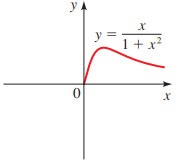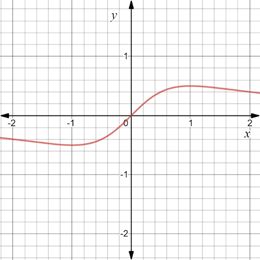# To graph : The equation y = x 1 + x 2 such that it is symmetric with respect to y-axis.### Precalculus: Mathematics for Calcu...

6th Edition
Stewart + 5 others
Publisher: Cengage Learning
ISBN: 9780840068071### Precalculus: Mathematics for Calcu...

6th Edition
Stewart + 5 others
Publisher: Cengage Learning
ISBN: 9780840068071

#### Solutions

Chapter 1.8, Problem 85E
To determine

## To graph: The equation y=x1+x2 such that it is symmetric with respect to y-axis.Expert Solution

### Explanation of Solution

Given information:

The graph of the equation y=x1+x2 .Graph:

The graph of the equation y=x1+x2 such that it is symmetric with respect to originis provided below,Interpretation:

Graph of the equation is symmetric with respect to originmeans that graph is not changed when rotated 180° about the origin.

The equation is symmetric with respect to origin, when y is replaced by y and x is replaced by x , the equation remains unchanged.

### Have a homework question?

Subscribe to bartleby learn! Ask subject matter experts 30 homework questions each month. Plus, you’ll have access to millions of step-by-step textbook answers!